Courses

# Physics - 2014 Past Year Paper

## 35 Questions MCQ Test IIT JAM Physics Past Year Papers | Physics - 2014 Past Year Paper

Description
This mock test of Physics - 2014 Past Year Paper for IIT JAM helps you for every IIT JAM entrance exam. This contains 35 Multiple Choice Questions for IIT JAM Physics - 2014 Past Year Paper (mcq) to study with solutions a complete question bank. The solved questions answers in this Physics - 2014 Past Year Paper quiz give you a good mix of easy questions and tough questions. IIT JAM students definitely take this Physics - 2014 Past Year Paper exercise for a better result in the exam. You can find other Physics - 2014 Past Year Paper extra questions, long questions & short questions for IIT JAM on EduRev as well by searching above.
QUESTION: 1

Solution:
QUESTION: 2

Solution:
QUESTION: 3

### A conducting slab of copper PQRS is kept on the xy plane in a uniform magnetic field along xaxis as indicated in the figure. A steady current I flows through the cross- section of the slab along the y- axis. The direction of the electric field inside the slab, arising due to the applied magnetic field is along the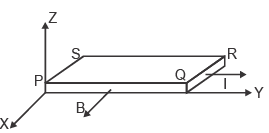Solution:
QUESTION: 4

A collimated beam of light of diameter 1 mm is propagating along the x- axis. The beam is to be expanded 10 mm using a combination of two convex lenses. A lens of focal length of 50 mm and another lens with focal length F are to be kept at a distance d between them. The values of F and d respectively, are

Solution:
QUESTION: 5

Octal equivalent of decimal number 47810 is

Solution:
QUESTION: 6

A spherical ball of ice has radius R0 and is rotating with an angular speed w about an axis passing through its centre. At time t = 0, it starts acquiring mass because the moisture around it starts to freeze on it uniformly. As a result its radius increases as R(t) = R0 + αt, where a is a constant.
The curve which best describes its angular speed with time is

Solution:
QUESTION: 7

In a photoelectric effect experiment, ultraviolet light of wavelength 320 nm falls on the photocathode with work function of 2.1 eV. The stopping potential should be close to

Solution:
QUESTION: 8

In 1- dimension, an ensemble of N classical particles has energy of the form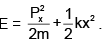The average internal energy of the system at temperature T is ___ .

Solution:
QUESTION: 9

In an ideal operational amplifier depicted below, the potential at node A is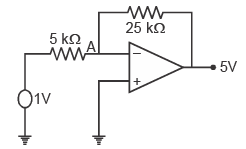Solution:
QUESTION: 10

To operate a npn transistor in active region, its emitter- base and collector- base junction respectively, should be

Solution:
QUESTION: 11

The value of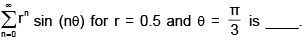Solution:
QUESTION: 12

In a parallel plate capacitor the distance between the plates is 10 cm. Two dielectric slabs of thick- ness 5 cm each and dielectric constants K1= 2 and K2 = 4 respectively, are inserted between the plates. A potential of 100 V is applied across the capacitor as shown in the figure. The value of the net bound surface charge density at the interface of the two dielectrics is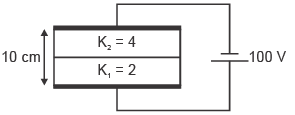Solution:
QUESTION: 13

The electric fields of two light sources with nearby frequencies ω1 and ω2, and wave vectors k1 and k2, are expressed as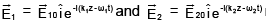respectively. The interference pattern on the screen is photographed at t = t0; denote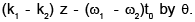For this pattern

Solution:
QUESTION: 14

A solid metallic cube of heat capacity S is at temperature 300 K. It is brought in contact with a reservoir at 600 K. If the heat transfer takes place only between the reservoir and the cube, the entropy change of the reservoir after reaching the thermal equilibrium is ____

Solution:
QUESTION: 15

If the surface integral of the field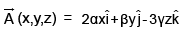over the closed surface of an arbitrary unit sphere is to be zero, then the relationship between α, β  and γ is

Solution:
QUESTION: 16

The moment of inertia of a disc about one of its diameters is IM. The mass per unit area of the disc is proportional to the distance from its centre. If the radius of the disc is R and its mass is M, the value of IM is _______

Solution:
QUESTION: 17

The rigid uniform horizontal wire PQ of mass M, pivoted at P, carries a constant current I. It rotates with a constant angular speed in a uniform vertical magnetic field B. If the current were switched off, the angular acceleration of the wire, in terms of B, M and I would be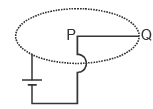Solution:
QUESTION: 18

Two points N and S are located in the northern and southern hemisphere, respectively, on the same longitude. Projectiles P and Q are fired from N and S, respectively, towards each other.Which of the following option is correct for the projectiles as they approach the equator ?

Solution:
QUESTION: 19

Two particles A and B of mass m and one particle C of mass are kept on the x axis in thr order ABC, Particle A is given a velocity vi . Consequently there are two collisions, both of which are completely inelastic. If the net energy loss because of these collisions is 7/8 of the initial energy,, the value of M is (ignore frictional losses)

Solution:
QUESTION: 20

The line integral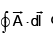of a vector field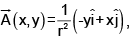where r2 = x2 + y2, is taken around a square (see figure) of side unit length and centered at (x0, y0) with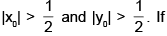the value of the integral is L, then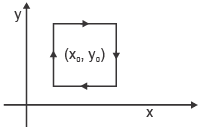Solution:
QUESTION: 21

Diamond lattice can be considered as a combination of two fcc lattices displaced along the body diagonal by one quarter of its length. There are eight atoms per unit cell. The packing fraction of the diamond structure is

Solution:
QUESTION: 22

Thermal neutrons (energy = 300 kB = 0.025 eV) are sometimes used for structural determination of materials. The typical lattice spacing of a material for which these can be used is

Solution:
QUESTION: 23

What is the maximum height above the dashed line attained by the water stream coming out at B from a thin tube of the water tank assembly shown in the figure? Assume h = 10 m, L = 2m, and θ = 30o.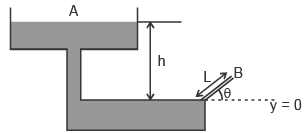Solution:
QUESTION: 24

A steady current in a straight conducting wire produces a surface charge on it. Let Eout and Ein be the magnitudes of the electric fields just outside and just inside the wire, respectively. W hich of the following statements is true for these fields ?

Solution:
QUESTION: 25

A small charged spherical shell of radius 0.01 m is at a potential of 30 V. The electrostatic energy of the shell is

Solution:
QUESTION: 26

At an instant shown, three point masses m, 2m and 3m rest on a horizontal surface, and are at the vertices of an equilateral triangle of unit side length. Assuming that G is the gravitational constant, the magnitude and direction of the torque on the mass 3m, about the point O, at that instant is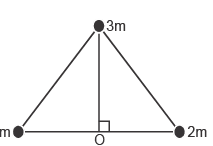Solution:
QUESTION: 27

A sine wave of 5V amplitude is applied at the input of the circuit shown in the figure. which of the following waveforms represents the output most closely?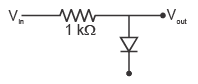Solution:
QUESTION: 28

1011 binary have been applied at X3 X2 X1 X0 input in the shown logic circuit made of XOR gates. The binary output Y3 Y2 Y1 Y0 of the circuit will be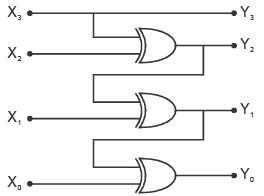Solution:
QUESTION: 29

A ring of radius R carries a linear charge density l.  It is rotating with angular speed w.  The magnetic field at its center is

Solution:
QUESTION: 30

A stationary source (see figure below) emits sound waves of frequency f towards a wall. If an observer moving with speed u in a direction perpendicular to the wall, measures a frequency f’ = 9/8 f at the instant shown, then u is related to the speed of sound Vs as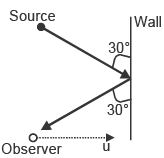Solution:
QUESTION: 31

A real gas has specific volume v at temperature T. Its coefficient of volume expansion and isothermal compressibility are a and kT, respectively. Its molar specific heat at constant pressure Cp and molar specific heat at constant volume Cv are related as

Solution:
QUESTION: 32

Two frames, O and O’, are in relative motion as shown. O’ is moving with speed c/2, where c is the speed of light. In frame O, two separate events occur at (x1, t1) and (x2, t2). In frame O’, these events occur simultaneously. The value of (x2 - x1)(t2 - t1) is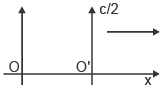Solution:
QUESTION: 33

White light is incident on a grating G1 with groove density 600 lines/mm and width 50 mm. A small portion of the diffracted light is incident on another grating G2 with groove density 1800 lines/mm and width 15 mm. The resolving power of the combined system is

Solution:
QUESTION: 34

Four particles of mass m each are inside a two dimensional square box of side L. If each state obtained from the solution of the Schrodinger equation is occupied by only one particle, the minimum energy of the system in units of  h2/mL2 is

Solution:
QUESTION: 35

At atmospheric pressure (= 105 Pa), aluminium melts at 550 K. As it melts, its density decreases from 3 x 103 kg/m3 to 2.9 x 103 kg/m3. Latent heat of fusion of aluminium is 24 x 103 J/kg. The melting point of aluminium at a pressure of 107 Pa is closest to

Solution: